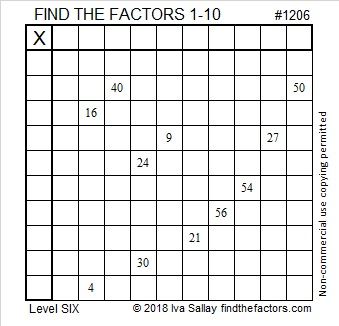# 1206 and Level 6

If you carefully study all the clues in this Level 6 puzzle and use logic, you should be able to solve the puzzle. Stick with it and you’ll succeed!Print the puzzles or type the solution in this excel file: 10-factors-1199-1210

Here are some facts about the number 1206:

• 1206 is a composite number.
• Prime factorization: 1206 = 2 × 3 × 3 × 67, which can be written 1206 = 2 × 3² × 67
• The exponents in the prime factorization are 1, 2, and 1. Adding one to each and multiplying we get (1 + 1)(2 + 1)(1 + 1) = 2 × 3 × 2 = 12. Therefore 1206 has exactly 12 factors.
• Factors of 1206: 1, 2, 3, 6, 9, 18, 67, 134, 201, 402, 603, 1206
• Factor pairs: 1206 = 1 × 1206, 2 × 603, 3 × 402, 6 × 201, 9 × 134, or 18 × 67,
• Taking the factor pair with the largest square number factor, we get √1206 = (√9)(√134) = 3√134 ≈ 34.72751Notice that 6·201 = 1206. Not very many numbers can equal themselves by using their own digits in a different way with +, -, ×, ÷, and/or parenthesis. That fact makes 1206 only the seventeenth Friedman Number.

1206 is also the sum of the twenty prime numbers from 19 to 103.

This site uses Akismet to reduce spam. Learn how your comment data is processed.